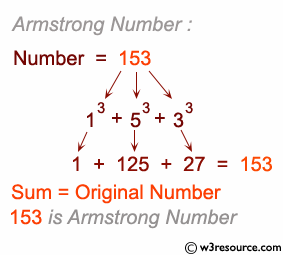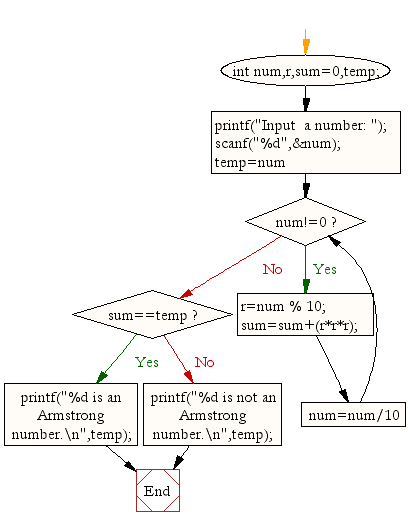﻿ C : Check if a given number is an armstrong number or not# C Exercises: Check whether a given number is an armstrong number or not

## C For Loop: Exercise-29 with Solution

Write a C program to check whether a given number is an armstrong number or not.

When the sum of the cube of the individual digits of a number is equal to that number, the number is called Armstrong number. For Example 153 is an Armstrong number because 153 = 13+53+33.

Test Data :
Input a number: 153
Expected Output :
153 is an Armstrong number.

Pictorial Presentation:## Visualize C code execution:

The following tool visualize what the computer is doing step-by-step as it executes the said program:

Sample Solution:

C Code:

``````#include <stdio.h>

void main(){
int num,r,sum=0,temp;

printf("Input  a number: ");
scanf("%d",&num);

for(temp=num;num!=0;num=num/10){
r=num % 10;
sum=sum+(r*r*r);
}
if(sum==temp)
printf("%d is an Armstrong number.\n",temp);
else
printf("%d is not an Armstrong number.\n",temp);

}
```
```

Sample Output:

```Input  a number: 153
153 is an Armstrong number.
```

Flowchart:C Programming Code Editor:

Improve this sample solution and post your code through Disqus.

What is the difficulty level of this exercise?

Test your Programming skills with w3resource's quiz.

﻿

## C Programming: Tips of the Day

What is the size of an enum in c?

An enum is only guaranteed to be large enough to hold int values. The compiler is free to choose the actual type used based on the enumeration constants defined so it can choose a smaller type if it can represent the values you define. If you need enumeration constants that don't fit into an int you will need to use compiler-specific extensions to do so.

Ref : https://bit.ly/3ypg4k8